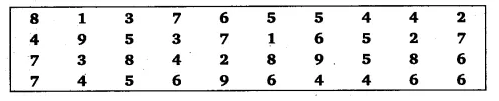# In a Mathematics test, the following marks were obtained by 40 students

In a Mathematics test, the following marks were obtained by 40 students. Arrange these marks in a table using tally marks.(a) Find how many students obtained marks equal to or more than 7?
(b) How many students obtained marks below 4?